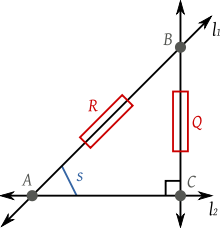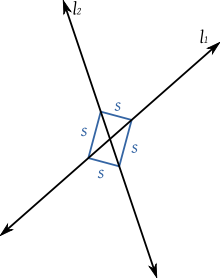# Rational trigonometry

﻿
Rational trigonometry

Rational trigonometry is a recently introduced approach to trigonometry that eschews all transcendental functions (such as sine and cosine) and all proportional measurements of angles. In place of angles, it characterizes the separation between lines by a quantity called the "spread", which is a rational function of their slopes. In place of the classical transcendental functions, it introduces a number of "laws" between the lengths and spreads of lines bound by various geometric configurations, notably triangles.

Rational trigonometry was introduced by Prof. Norman J. Wildberger in his 2005 book Divine Proportions: Rational Trigonometry to Universal Geometry. The book is purposefully critical of traditional mathematics. One of the main claims the author makes is that the field of trigonometry is being taught and applied using choices in measurements and relations (such as angles and transcendental functions) that are arbitrarily complicated, are not necessary in order to reason about most concepts of the field, nor are they needed for most practical applications.

The author proposes new basic measurements on which to base the field itself and related applications (namely, the square of distance instead of the plain distance between two points, and the spread instead of the angle between two lines) and goes on to prove an array of theorems and laws to work with these measurements.

From there, the author rebuilds a great part of Euclidean geometry on top of his new proposed measurements, using only rational equivalences, which allow him to avoid any assumptions about the underlying scalar field. This is the Universal Geometry part of the book, where most classical findings of geometry are shown to be applicable to any field, including the field of rational numbers or finite fields.

Wildberger holds a Ph.D. in mathematics from Yale University, and taught at Stanford University from 1984 to 1986 and at the University of Toronto from 1986 to 1989; he is currently an associate professor of mathematics at the University of New South Wales, Australia.

Instead of distance and angle, rational trigonometry uses as its fundamental units quadrance (square of distance) and spread (square of sine of angle). This choice of variables enables calculations to produce output results whose complexity matches that of the input data. For instance, in a typical trigonometry problem if rational numbers are assigned to all quadrances and spreads, then the calculated results will be rational numbers (or roots of rational numbers).

This rationality is obtained at the expense of linearity. Unlike the traditional distance and angle units, doubling or halving a quadrance or spread does not double or halve a length or a rotation. Similarly, the sum of two lengths or rotations is not the sum of their individual quadrances or spreads.

For distinction, Wildberger refers to the traditional trigonometry as classical trigonometry. It is otherwise broadly based on Cartesian analytic geometry, with a point defined as an ordered pair (x, y) and a line as a general linear equation$Ax + By + C = 0.\,$

The mathematics of rational trigonometry is, applications aside, a special instance of the description of geometry in terms of linear algebra (using rational methods such as dot products and quadratic forms), but students who are first learning trigonometry are often not taught about the use of linear algebra in geometry. Changing this state of affairs is a stated aim of Wildberger's book (to paraphrase his comments).

## Trigonometry over arbitrary fields

Rational trigonometry makes it possible to do trigonometry over any field, not just the field of real numbers.

The quadrance is the square of the distance.

Quadrance and distance are concerned with the separation of points. Quadrance differs from standard distance in that it squares the distance. Most immediately, this means that calculating the distance (or, more accurately, quadrance) between two points in 2-dimensional space is easier, as there is no need to find the square root of the sum of the squares of the differences in the x and y coordinates.

In the (xy)-plane, the quadrance Q(A1A2) for the points A1 and A2 is defined as$Q(A_1, A_2) = (x_2 - x_1)^2 + (y_2 - y_1)^2.\,$Suppose  1 and  2 intersect at the point A. Let C be the foot of the perpendicular from B to  2. Then the spread is s = Q/R.The spread of two lines can be measured in four equivalent positions.

Spread, a measure of the separation of lines, is a dimensionless number in the range [0, 1]. The value of the spread is the square of the sine of the angle.

Suppose two lines,  1 and  2, intersect at the point A as shown at right. Choose a point B ≠ A on  1 and let C be the foot of the perpendicular from B to  2. Then the spread s is$s(\ell_1, \ell_2) = \frac{Q(B, C)}{Q(A, B)} = \frac{Q}{R}.$

In rational trigonometry, spread is a fundamental concept, somewhat but not precisely corresponding to the concept in traditional geometry of angle. Spread describes a relationship between two lines, whereas angle describes a relationship between two rays emanating from a common point.

The spread is the square of the sine of the angle.

0 0 0
30 (1/6)π 1/4
45 (1/4)π 1/2
60 (1/3)π 3/4
90 (1/2)π 1
120 (2/3)π 3/4
135 (3/4)π 1/2
150 (5/6)π 1/4
180 π 0

Spread is not proportional to degrees or radians, and has a period of 180 degrees (π radians).

## Laws of rational trigonometry

Wildberger states that there are five basic laws in rational trigonometry and these laws can be easily verified using high-school level mathematics. Some are equivalent to standard trigonometrical formulae with the variables expressed as quadrance and spread.

The three points A1A2A3,  are collinear precisely when:$(Q_{1} + Q_{2} + Q_{3})^2 = 2(Q_{1}^{2} + Q_{2}^{2} + Q_{3}^{2}).\,$

This is equivalent to using Heron's formula, the condition for collinearity being that the triangle formed by the three points has zero area.

It can either be proved by analytic geometry (the preferred means within rational trigonometry) or derived from Heron's formula, using the condition for collinearity that the triangle formed by the three points has zero area.

### Pythagoras' theorem

The lines A1A3 and A2A3 are perpendicular precisely when:$Q_1 + Q_2 = Q_3.\,$

This is equivalent to the Pythagorean theorem.

There are many classical proofs of Pythagoras' theorem; this one is framed in the terms of rational trigonometry.

The spread of an angle is the square of its sine. Given the triangle ABC with a spread of 1 between sides AB and AC,$Q(AB) + Q(AC) = Q(BC)\,$

where Q is the "quadrance", i.e. the square of the distance.

For any triangle$\overline{A_{1} A_{2} A_{3}}$ with non zero quadrances:$\frac{s_{1}}{Q_{1}}=\frac{s_{2}}{Q_{2}}=\frac{s_{3}}{Q_{3}}.\,$

This is analogous to the law of sines.

### Cross law

For any triangle$\overline{A_{1} A_{2} A_{3}}$ define the cross c3 as c3 = 1 − s3. Then:$(Q_1 + Q_2 - Q_3)^2 = 4Q_1 Q_2 c_3.\,$

This is analogous to the law of cosines.

For any triangle$\overline{A_1 A_2 A_3},$$(s_1 + s_2 + s_3)^2 = 2(s_1^2 + s_2^2 + s_3^2) + 4s_1 s_ 2 s_ 3 .\,$

This corresponds (roughly) to the angle sum formulae for sine and cosine.

Given the coordinates of two points (x1y1) and (x2y2), the quadrance between them is$Q = (x_2 -x_1)^2 + (y_2 - y_1)^2.\,$

Given two lines whose equations are a1x + b1y = constant and a2x + b2y = constant, the spread between them is$s = \frac{(a_1 b_2 - a_2 b_1)^2}{(a_1^2 + b_1^2)(a_2^2 + b_2^2)}.\,$

## Ease of calculation

Rational trigonometry makes some problems solvable with only addition, subtraction, multiplication, and division, with fewer uses of other functions such as square roots, sine, and cosine (compared with classical trigonometry). Such algorithms execute more efficiently on most computers, for problems such as solving triangles. Other computations, such as computing the quadrance of a line segment given the quadrance of two collinear line segments which compose it do involve more computations than their classical equivalents.